Hostname: page-component-7d684dbfc8-mqbnt Total loading time: 0 Render date: 2023-09-27T23:15:25.907Z Has data issue: false Feature Flags: { "corePageComponentGetUserInfoFromSharedSession": true, "coreDisableEcommerce": false, "coreDisableSocialShare": false, "coreDisableEcommerceForArticlePurchase": false, "coreDisableEcommerceForBookPurchase": false, "coreDisableEcommerceForElementPurchase": false, "coreUseNewShare": true, "useRatesEcommerce": true } hasContentIssue false

# The Central Limit Theorem for Subsequences in Probabilistic Number Theory

Published online by Cambridge University Press:  20 November 2018

## Abstract

Core share and HTML view are not possible as this article does not have html content. However, as you have access to this content, a full PDF is available via the ‘Save PDF’ action button.

Let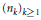${{\left( {{n}_{k}} \right)}_{k\ge 1}}$ be an increasing sequence of positive integers, and let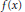$f\left( x \right)$ be a real function satisfying

1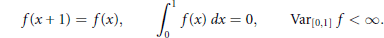$$f\left( x+1 \right)=f\left( x \right),\int\limits_{0}^{1}{f\left( x \right)}dx=0,\text{Va}{{\text{r}}_{\left[ 0,1 \right]}}f<\infty$$

If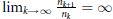${{\lim }_{k\to \infty }}\frac{{{n}_{k+1}}}{{{n}_{k}}}=\infty$ the distribution of

2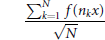$$\frac{\sum\nolimits_{k=1}^{N}{f\left( {{n}_{k}}x \right)}}{\sqrt{N}}$$

converges to a Gaussian distribution. In the case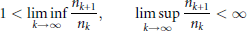$$1<\underset{k\to \infty }{\mathop{\lim \inf }}\,\,\frac{{{n}_{k+1}}}{{{n}_{k}}},\,\,\underset{k\to \infty }{\mathop{\text{lim}\,\text{sup}}}\,\,\frac{{{n}_{k+1}}}{{{n}_{k}}}<\infty$$

there is a complex interplay between the analytic properties of the function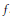$f$, the number-theoretic properties of${{\left( {{n}_{k}} \right)}_{k\ge 1}}$, and the limit distribution of (2).

In this paper we prove that any sequence${{\left( {{n}_{k}} \right)}_{k\ge 1}}$ satisfying$\lim {{\sup }_{k\to \infty }}{{n}_{k+1}}/{{n}_{k}}=1$ contains a nontrivial subsequence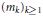${{\left( {{m}_{k}} \right)}_{k\ge 1}}$ such that for any function satisfying (1) the distribution of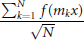$$\frac{\sum\nolimits_{k=1}^{N}{f\left( {{m}_{k}}x \right)}}{\sqrt{N}}$$

converges to a Gaussian distribution. This result is best possible: for any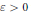$\varepsilon >0$ there exists a sequence${{\left( {{n}_{k}} \right)}_{k\ge 1}}$ satisfying lim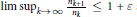$\underset{k\to \infty }{\mathop{\sup }}\,\frac{{{n}_{k+1}}}{{{n}_{k}}}\le 1+\varepsilon$ such that for every nontrivial subsequence${{\left( {{m}_{k}} \right)}_{k\ge 1}}$ of${{\left( {{n}_{k}} \right)}_{k\ge 1}}$ the distribution of (2) does not converge to a Gaussian distribution for some$f$.

Our result can be viewed as a Ramsey type result: a sufficiently dense increasing integer sequence contains a subsequence having a certain requested number-theoretic property.

Type
Research Article
Information
Canadian Journal of Mathematics , 01 December 2012 , pp. 1201 - 1221

## References

 Aistleitner, C., Diophantine equations and the LIL for the discrepancy of sublacunary sequences. Illinois J. Math. 53(2009), 785815.Google Scholar
 Aistleitner, C., On the law of the iterated logarithm for the discrepancy of Lacunary sequences. Trans. Amer. Math. Soc. 362(2010), 59675982. http://dx.doi.org/10.1090/S0002-9947-2010-05026-3 Google Scholar
 Aistleitner, C. and Berkes, I., On the central limit theorem for f (nkx). Probab˙Theory Related Fields 146(2010), 267289. http://dx.doi.org/10.1007/s00440-008-0190-6 Google Scholar
 Berkes, I., A central limit theorem for trigonometric series with small gaps. Z.Wahrsch. Verw. Gebiete 47(1979), 157161. http://dx.doi.org/10.1007/BF00535280 Google Scholar
 Berkes, I., Philipp, W. and Tichy, R. F., Empirical processes in probabilistic number theory: the LIL for the discrepancy of (nk!) mod 1. Illinois J. Math. 50(2006), 107145 (electronic).Google Scholar
 Berkes, I., Metric discrepancy results for sequences ﹛nkx﹜ and Diophantine equations. In: Diophantine approximation, Dev. Math. 16, Springer Wien New York, Vienna, 2008, 95105.Google Scholar
 Bobkov, S. G. and Götze, F., On the central limit theorem along subsequences of noncorrelated observations. Teor. Veroyatnost. i Primenen. 48(2003), 745765.Google Scholar
 Bobkov, S. G. and Götze, F., Concentration inequalities and limit theorems for randomized sums. Probab. Theory Related Fields 137(2007), 4981. http://dx.doi.org/10.1007/s00440-006-0500-9 Google Scholar
 Burkill, H. and Mirsky, L., Monotonicity. J. Math. Anal. Appl. 41(1973), 391410. http://dx.doi.org/10.1016/0022-247X(73)90214-X Google Scholar
 Chatterji, S. D., A principle of subsequences in probability theory: the central limit theorem. Advances in Math. 13(1974), 3154; correction, ibid. 14(1974), 266–269. http://dx.doi.org/10.1016/0001-8708(74)90064-4 Google Scholar
 Drmota, M. and Tichy, R. F., Sequences, discrepancies and applications. Lecture Notes in Math. 1651, Springer–Verlag, Berlin, 1997.Google Scholar
 Dupain, Y. and Sós, V. T., On the one-sided boundedness of discrepancy-function of the sequence ﹛n﹜. Acta Arith. 37(1980), 363374.Google Scholar
 Erdös, P. and Gál, I. S., On the law of the iterated logarithm. I, II. Nederl. Akad.Wetensch. Proc. Ser. A 58 = Indag. Math. 17(1955), 6576, 77–84.Google Scholar
 Fukuyama, K., A central limit theorem for trigonometric series with bounded gaps. Probab. Theory Related Fields 149(2011), 139148. http://dx.doi.org/10.1007/s00440-009-0245-3 Google Scholar
 Fukuyama, K., A central limit theorem and a metric discrepancy result for sequences with bounded gaps. In: Dependence in Probability, Analysis and Number Theory, Kendrick Press, 2010, 233246.Google Scholar
 Fukuyama, K. and Nakata, K., A metric discrepancy result for the Hardy–Littlewood–Pólya sequences. Monatsh. Math. 160(2010), 4149. http://dx.doi.org/10.1007/s00605-008-0051-5 Google Scholar
 Fukuyama, K. and Petit, B., Le théorème limite central pour les suites de R. C. Baker. Ergodic Theory Dynam. Systems 21(2001), 479492.Google Scholar
 Gapoškin, V. F., Lacunary series and independent functions. Uspehi Mat. Nauk 21(1966), 382.Google Scholar
 Gapoškin, V. F., The central limit theorem for certain weakly dependent sequences. Teor. Verojatnost. i Primenen. 15(1970), 666684.Google Scholar
 Kac, M., Probability methods in some problems of analysis and number theory. Bull. Amer. Math. Soc. 55(1949), 641665. http://dx.doi.org/10.1090/S0002-9904-1949-09242-X Google Scholar
 Kesten, H., The discrepancy of random sequenceskx﹜. Acta Arith. 10(1964/1965), 183213.Google Scholar
 Kuipers, L. and Niederreiter, H., Uniform distribution of sequences. Pure and Applied Mathematics, Wiley-Interscience [John Wiley & Sons], New York, 1974.Google Scholar
 Něsetřil, J., Ramsey theory. In: Handbook of combinatorics, Vol. 1, 2, Elsevier, Amsterdam, 1995, 13311403.Google Scholar
 Philipp, W., Limit theorems for lacunary series and uniform distribution mod1. Acta Arith. 26(1974/75), 241251.Google Scholar
 Philipp, W., Empirical distribution functions and strong approximation theorems for dependent random variables. A problem of Baker in probabilistic number theory. Trans. Amer. Math. Soc. 345(1994), 705727. http://dx.doi.org/10.2307/2154995 Google Scholar
 Salem, R. and Zygmund, A., On lacunary trigonometric series. Proc. Nat. Acad. Sci. U. S. A. 33(1947), 333338. http://dx.doi.org/10.1073/pnas.33.11.333 Google Scholar
 Salem, R. and Zygmund, A., La loi du logarithme itéré pour les séries trigonométriques lacunaires. Bull. Sci. Math. (2) 74(1950), 209224.Google Scholar
 Sanders, T., On Roth's theorem on progressions. Ann. of Math. 174(2011), 619636. http://dx.doi.org/10.4007/annals.2011.174.1.20 Google Scholar
 Schoissengeier, J., On the discrepancy of (n). Acta Arith. 44(1984), 241279.Google Scholar
 Schoissengeier, J., A metrical result on the discrepancy of (n). Glasgow Math. J. 40(1998), 393425. http://dx.doi.org/10.1017/S0017089500032742 Google Scholar
 Szemerédi, E., On sets of integers containing no k elements in arithmetic progression. Acta Arith. 27(1975), 199245.Google Scholar
 Takahashi, S., A gap sequence with gaps bigger than the Hadamards. Tôhoku Math. J. 13(1961), 105111. http://dx.doi.org/10.2748/tmj/1178244355 Google Scholar
 Zygmund, A., Trigonometric series. Vol. I, II. Cambridge Mathematical Library, Cambridge University Press, Cambridge, 1988. Reprint of the 1979 edition.Google Scholar